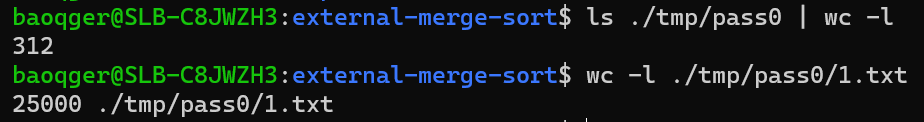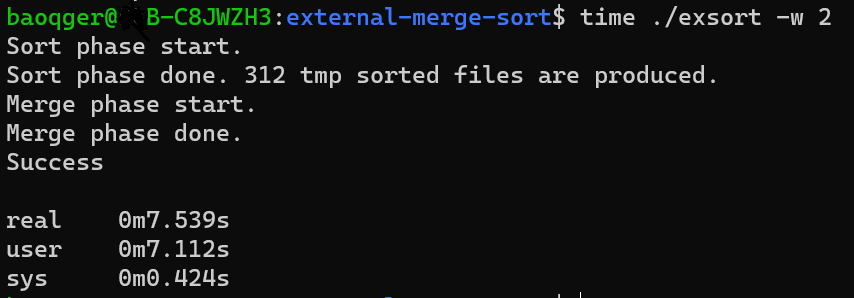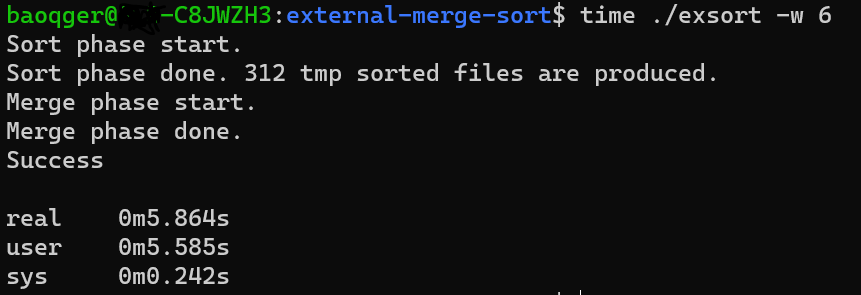# External Mergesort: part two

### Introduction

In this last article, we examined how external mergesort works in theory, let’s implement it now.

First, you can find all the source codes of this implementation in this Github repo. In this repo, I implemented both two-way and multi-way solutions, which are tracked in different branches, please be aware of this. But for the sake of simplicity, I will focus on the generalized multi-way mergesort solution in this article.

### Data Preparation

Before diving into the code, let’s define the problem we need to solve here. I will generate an input file containing several millions of seven digits random numbers, from 1,000,000 to 9,999,999. The random numbers can be duplicated, and each number is stored in one new line of the input file. The input file can be prepared with the following Bash script which calls GNU shuf :

The generated input file is roughly 60 MB in size. For modern computers, it can be loaded to memory easily. But since we are working external memory algorithm, so let’s assume we are running the algorithm on an old computer, which has only 100000 Byte memory. Based on this assumed restriction, we need to sort the numbers in the input file and save the result in a new file.

### Implementation

Let’s define some global constants in this algorithm:

MEMORY_LIMIT denotes the 100000 bytes memory limit; In C, we can use the type unsigned int to store an integer in the range from 1,000,000 to 9,999,999. So RECORD_SIZE means each record(or integer) will take up 4 bytes of memory.

And by default, the algorithm will use the two-way merge, but the user can pass an argument to run a multi-way merge too.

##### Sort phase

The sort phase is implemented inside the separationSort function as follows:

The logic is not difficult. The function takes the input file descriptor as a parameter and reads each line(via the getline method) in a loop until reaches the end of the file. The numbers will be read into the memory buffer before hitting the memory limit. When the memory buffer is full(100000 bytes), the numbers are sorted with the function mergeSort.

The function mergeSort is defined inside the file internal_sort.c, which implements the classic internal merge sorting algorithm. I will not cover it in this article, since you can find many documents about it online. If you don’t know about it, please spend some time learning about it. Of course, you can replace it with other sorting algorithms like quick sort too. I leave this for the readers.

After sorting, the numbers are saved in the temporary files in the directory of ./tmp/pass0. The filename is just the run number.

We can verify the result of the sort phase as follows:You can see each file contains up to 25000 (equal to MEMORY_LIMIT/RECORD_SIZE) numbers and 312 files are created in pass0.

Note that I will not examine the details about how to make a new directory and how to open a new file to read or write. You can learn such Linux file I/O concepts by yourself.

##### Merge phase

The exMerge function controls the passes in the merge phase starting from pass1.

The variable fileNum stores the run number in each pass. And the variable ways denotes the number of multi-way. Thus, the run number of the next pass should be calculated as fileNum / ways.

The detailed merging logic is inside the function exMergeSort, which takes two parameters. pass means the current pass number(starting from 1), while nums means how many runs(or sub-files) in the last pass need to be merged.

The above code creates a temp directory for each pass and a temp file for each run.

Next, we create an array of the input files for each run. And the input file is inside the temp directory of the last pass. Each run merges multiple files in the for loop. The only trick logic here is that the remaining files in the last run may be less than the number of ways declared, we need to handle that properly (line 5 of the below code block).

Next, we need to read one number from every input file until only one file is not run out. Find the smallest one and save it in the temp output run file. And for the last remaining file, remember to put the rest numbers into the output file too.

The above code bock utilizes several methods like getRecord, validateRecords, getMinRecordIndex and getLastRemainRecordIndex as follows, and these functions are easy to understand.

In detail, you can refer to the source code of this github repo. Next, let’s evaluate the performance of this algorithm by tuning the number of ways for merging.

### Performance Evaluation

We’ll use the Linux time utility to measure the running time of this algorithm.

The result of two-way mergesort is:while six-way mergesort can complete with a shorter runtime.